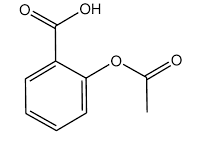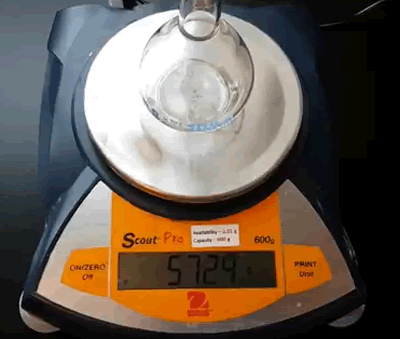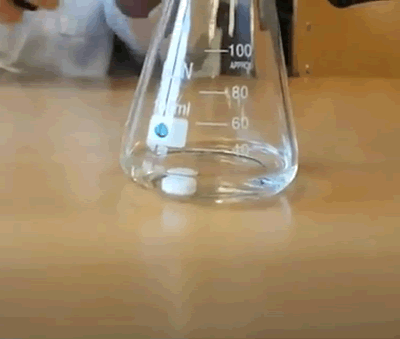Volumetric analysis -aspirin titration# acetylsalicylic acid

The following are steps involved in the analysis of the amount of acetylsalicylic acid in a tablet of Aspirin. Click on the blue text for information.
The objective is to measure the concentration of active ingredient in one tablet in a particular brand of Aspirin.
Step 1 Prepare a standard solution of KOH.

a. measure the mass of a 100 mL volumetric flask.
b. place approximately 0.70 g of KOH into the volumetric flask
c. reweigh the flask and KOH
d. record the mass of KOH in the volumetric flask.
e. fill the volumetric flask to the mark with distilled water.
f. calculate the amount, in mol, of KOH in the volumetric flask.
e. calculate the [KOH] in the volumetric flask.Step 2 Fill the burette with the standard solution and record the starting point of the meniscus. Record the starting point.Step 3 Weigh a tablet from the packet of Aspirin.

Record the mass of the tablet.Step 5 Place the tablet in a 100mL conical flask.

- add enough alcohol to completely dissolve the tablet.

- add two drops of phenolphthalein indicator to the 100 mL conical flask.

Step 6 Titrate the analyte in the conical flask with the standard solution until the end point is reached. Read the burette and record the result.1. What is the volume of the titre?

2. Calculate the mol of KOH delivered in the titre.

3. Given that acetylsalicylic acid is a monoprotic acid, calculate the mol of acid present in the conical flask.

4. Given the molar mass of acetylsalicylic acid is 180.2 g/mol calculate the mass of acetylsalicylic acid in the tablet.

5. Calculate the concentration of acetylsalicylic acid in the tablet in %m/m.

6. Is this consistent with the label?